nextnano.com  nextnano³  Download | Search | Copyright | Publications  * password protected nextnano³ software2D Core-Shell nanowire

# nextnano3 - Tutorial

## Schrödinger equation of a two-dimensional core-shell structure Hexagonal 2DEG - Two-dimensional electron gas in a delta-doped hexagonal shaped GaAs/AlGaAs nanowire heterostructure

Author: Stefan Birner

`==> 2DGaAs_AlGaAs_circle_nn3.in         / *_nnp.in - `input file for the nextnano3 and nextnano++ software``` ==> 2DGaAs_AlGaAs_hexagon_nn3.in        / *_nnp.in - ```input file for the nextnano3 and nextnano++ software``` ==> 2DGaAs_AlGaAs_hexagon_AlloySweep.in            - ```input file for the nextnano3 software``` ==> 2D_Hexagonal_Nanowire_2DEG_nn3.in   / *_nnp.in - ```input file for the nextnano3 and nextnano++ software

## Schrödinger equation of a two-dimensional core-shell structure

In this tutorial we solve the two-dimensional Schrödinger equation of a circular and a hexagonal GaAs/Al0.33Ga0.67As core-shell structure.

### Circular core-shell structure

`  ==> 2DGaAs_AlGaAs_circle.in`

The following figure shows the probability density of the 6th eigenstate of the circular GaAs/AlGaAs structure.
```Schroedinger_1band/2Dcb001_qc001_sg001_deg001_dir_ev006.fld ```Its energy level is higher than the AlGaAs barrier energy, i.e. this state is not confined in the circular shaped GaAs quantum well.
The horizontal and vertical slices are through the center and show the square of the probability amplitude of this eigenstate.The GaAs core has a radius of 5 nm. (It cannot be recognized on this plot.)
The AlGaAs shell has a radius of 15 nm. It is surrounded by an infinite barrrier which is defined by the circular quantum region of radius 15 nm (nextnano3 input file).
(Note: The nextnano++ input file cannot use a circular quantum region. Therefore a 15 nm x 15 nm square is used in this case as the quantum region. The circular confinement in this case comes from the "band offset" due to the surrounding material "air".)

### Hexagonal core-shell structure

`  ==> 2DGaAs_AlGaAs_hexagon.in`

The following figure shows the conduction band edge of the hexagonal GaAs/AlGaAs structure.
The GaAs region is indicated in black, the AlGaAs region in blue.
Horizontal and vertical slices through the center show the energy of the conduction band edge profile.
`band_structure/cb2D_001_sub001.fld`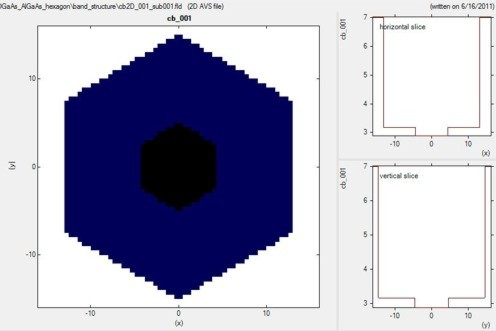The diameter of the hexagonal shaped GaAs core is ~8.66 nm (corresponding to an outer radius of the core of 5 nm).
The diameter of the hexagonal shaped AlGaAs shell is ~26 nm (corresponding to an outer radius of the shell of 15 nm).

The following figure shows the probability density of the 10th eigenstate of the circular GaAs/AlGaAs structure.
```Schroedinger_1band/2Dcb001_qc001_sg001_deg001_dir_ev010.fld ```Its energy level is higher than the AlGaAs barrier energy, i.e. this state is not confined in the hexagonal shaped GaAs quantum well.
The horizontal and vertical slices are through the center and show the square of the probability amplitude of this eigenstate.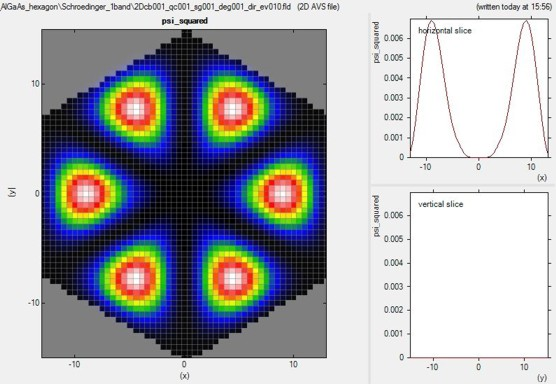The hexagonal GaAs core has an outer radius of 5 nm. It cannot be seen on this plot.
The AlGaAs shell has a diameter of 26 nm. It is surrounded by an infinite barrrier which is defined by the hexagonal shaped quantum region of diameter 26 nm (nextnano3 input file).
(Note: The nextnano++ input file cannot use a hexagonal quantum region. Therefore a 16 nm x 16 nm square is used in this case as the quantum region. The hexagonal confinement in this case comes from the "band offset" due to the surrounding material "air".)

### Alloy sweep

```  ==> 2DGaAs_AlGaAs_hexagon_AlloySweep.in       ```(There is no nextnano++ input file available that performs an alloy sweep as this feature is not supported in the input file. But you can use nextnanomat's Template feature to perform an alloy sweep with nextnano++.)

In the following, we vary the alloy content x of the ternary AlxGa1-xAs from 0 to 0.33 in 11 steps.

``` \$alloy-function   material-number             = 2   function-name               = constant ! ```spatially constant alloy content```   xalloy                      = 0.0d0    ! ```alloy content x```   alloy-sweep-active          = yes      ! ```to switch on alloy sweep```   alloy-sweep-step-size       = 0.03d0   ! ```increase alloy concentration in each step by 0.03```   alloy-sweep-number-of-steps = 11       ! ```number of alloy sweep steps

For x = 0, we have pure GaAs.
For x = 0.33 we have an AlGaAs/GaAs conduction band offset of 0.285 eV, and a valence band offset of -0.168 eV.
In the latter case, the quantum confinement is stronger.
Even for x = 0 we have "quantum confinement" due to the Dirichlet boundary conditions (corresponding to infinite barriers) at the shell surface that we use for the Schrödinger equation.
Consequently, even for x = 0, we get an e1-h1 transition energy from the lowest electron state (e1) to the highest heavy hole state (h1) that is larger than the band gap as shown in the following figure.The transition energies (e1-h1), as well as the spatial overlap intergral of the electron and hole ground state wave functions, are contained in this file:``` sweep_interband2D_qc001_vb001_sg001_cb001_sg001_deg001_dir.dat ```It contains:```   alloy      type                         el-hl[eV]     el[eV]        hl[eV]        matrix_element   0.330000   |<psi_vb001|psi_cb001_>|^2   1.522777615   2.965889676   1.443112062   0.936344908   0.300000   |<psi_vb001|psi_cb001_>|^2   1.520316794   2.963669699   1.443352905   0.931291593   ...```

The spatial overlap of electron and hole wave functions is always very high.
When there is only confinement due to the shell boundary, the matrix element is very high (99.8 %).
The matrix element must be smaller than 1 for x = 0 because the electron and hole masses are different.
The matrix element must be even smaller (94 %) for x = 0.33 (strong confinement) because in addition to the mass difference, the conduction and valence band offsets are not equivalent.
The matrix element has a minimum at around x = 0.06 because in this case the electron wave function penetrates into the barrier much stronger that the hole wave function does.
Thus the differences in well and barrier masses (as well as band offsets) play a high role for the spatial extension of the wave functions.

### Sweep over further variables

It is also possible to sweep over variables, like e.g. the outer radius of the GaAs core ("CoreOuterRadius") using nextnanomat's "Template" feature.
Just select "Range of values", choose the variable to be swept over, and specify a "from" "to" range, and the "step".
The click on "Create input files" and switch to the "Run" pane to start your batch list.
You can define your own variables using macros. Macros are described here.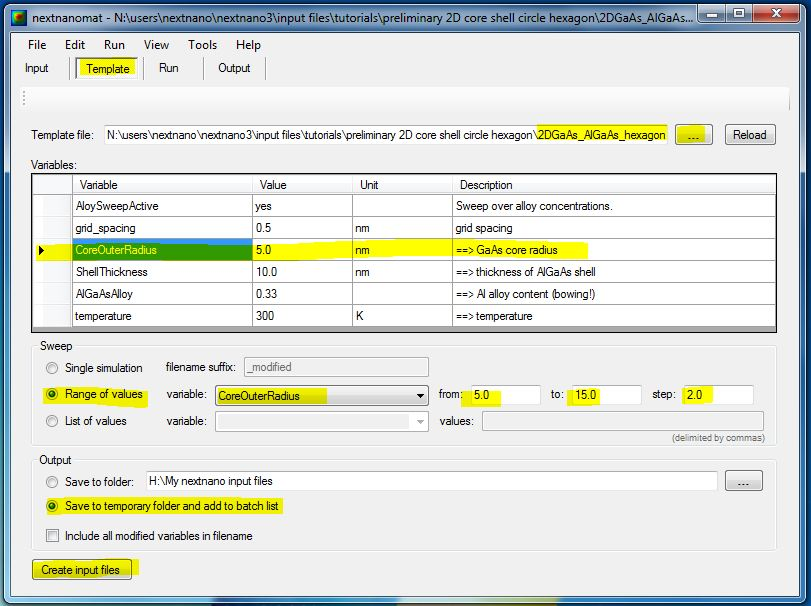## Hexagonal 2DEG - Two-dimensional electron gas in a delta-doped hexagonal shaped GaAs/AlGaAs nanowire heterostructure

`  ==> 2D_Hexagonal_Nanowire_2DEG.in`

The following example deals with a delta-doped GaAs/AlGaAs 2DEG (two-dimensional electron gas) structure.
In this case, the heterostructure consists of a hexagonal GaAs/AlGaAs nanowire.

The material layers are shown here in the following figure which shows the content of this file:` material_grid2D.fld`
`- `black: GaAs core
`- `blue: AlGaAs spacer
`- `green: Si-doped AlGaAs
`- `yellow: AlGaAs
`- `red: GaAs capping layer
`- ` white: Schottky barrier contact: The white layer itself is not included in the calculation. It only serves as a boundary condition.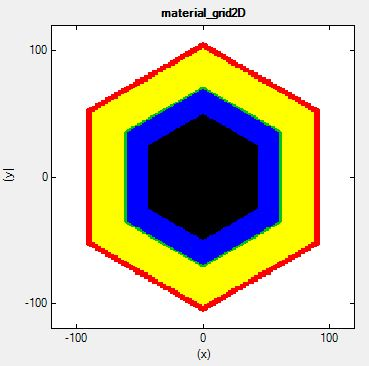Artist's view of a hexagonal nanowire. (The artist is 4 years old. ;-)

The self-consistently calculated conduction band edge (`band_structure/cb2D_Gamma_sub001.fld`) is shown next.
The horizontal and vertical slices through the center indicate the triangular potential well (conduction band minimum) where the 2DEG is located.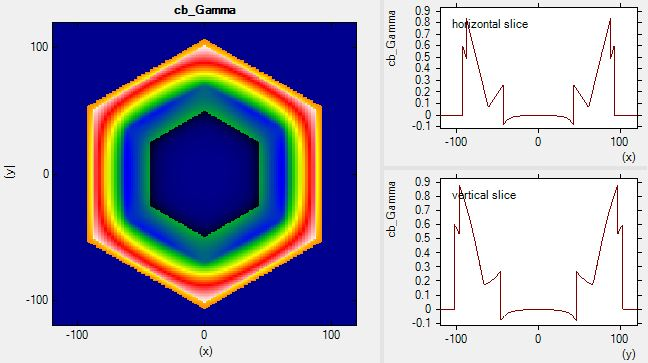The resulting 2DEG electron density (`densities/density2Del.fld`) is shown in the following figure.
At the corners, the electron density is significantly higher, thus one-dimensional conducting channels are formed.
Although the structure itself has a hexagonal symmetry, our rectangular grid breakes this symmetry.
Therefore the density in the upper/lower corner are different from the density at the left/right corners.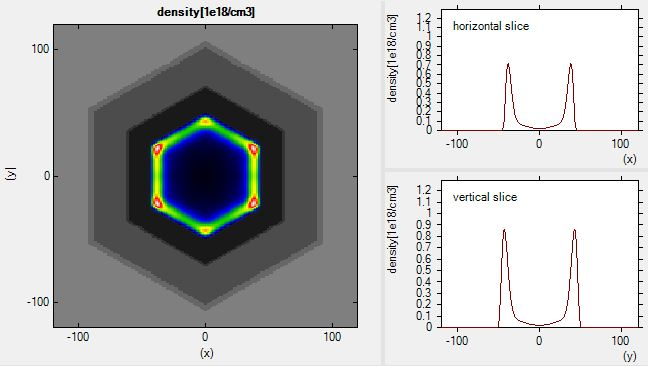The 2D Poisson equation and the 2D Schrödinger equation have been solved self-consistently.
The dimension of the Schroedinger matrix is 28,625.
The CPU time for this calculation was about 18 minutes.